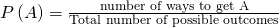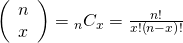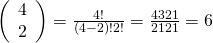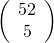Discrete Random Variables

# 20 Introduction

You can use probability and discrete random variables to calculate the likelihood of lightning striking the ground five times during a half-hour thunderstorm. (Credit: Leszek Leszczynski)A student takes a ten-question, true-false quiz. Because the student had such a busy schedule, he or she could not study and guesses randomly at each answer. What is the probability of the student passing the test with at least a 70%?

Small companies might be interested in the number of long-distance phone calls their employees make during the peak time of the day. Suppose the historical average is 20 calls. What is the probability that the employees make more than 20 long-distance phone calls during the peak time?

These two examples illustrate two different types of probability problems involving discrete random variables. Recall that discrete data are data that you can count, that is, the random variable can only take on whole number values. A random variable describes the outcomes of a statistical experiment in words. The values of a random variable can vary with each repetition of an experiment, often called a trial.

### Random Variable Notation

The upper case letter X denotes a random variable. Lower case letters like x or y denote the value of a random variable. If X is a random variable, then X is written in words, and x is given as a number.

For example, let X = the number of heads you get when you toss three fair coins. The sample space for the toss of three fair coins is TTT; THH; HTH; HHT; HTT; THT; TTH; HHH. Then, x = 0, 1, 2, 3. X is in words and x is a number. Notice that for this example, the x values are countable outcomes. Because you can count the possible values as whole numbers that X can take on and the outcomes are random (the x values 0, 1, 2, 3), X is a discrete random variable.

### Probability Density Functions (PDF) for a Random Variable

A probability density function or probability distribution function has two characteristics:

1. Each probability is between zero and one, inclusive.
2. The sum of the probabilities is one.

A probability density function is a mathematical formula that calculates probabilities for specific types of events, what we have been calling experiments. There is a sort of magic to a probability density function (Pdf) partially because the same formula often describes very different types of events. For example, the binomial Pdf will calculate probabilities for flipping coins, yes/no questions on an exam, opinions of voters in an up or down opinion poll, indeed any binary event. Other probability density functions will provide probabilities for the time until a part will fail, when a customer will arrive at the turnpike booth, the number of telephone calls arriving at a central switchboard, the growth rate of a bacterium, and on and on. There are whole families of probability density functions that are used in a wide variety of applications, including medicine, business and finance, physics and engineering, among others.

For our needs here we will concentrate on only a few probability density functions as we develop the tools of inferential statistics.

### Counting Formulas and the Combinational Formula

To repeat, the probability of event A , P(A), is simply the number of ways the experiment will result in A, relative to the total number of possible outcomes of the experiment.

As an equation this is:When we looked at the sample space for flipping 3 coins we could easily write the full sample space and thus could easily count the number of events that met our desired result, e.g. x = 1 , where X is the random variable defined as the number of heads.

As we have larger numbers of items in the sample space, such as a full deck of 52 cards, the ability to write out the sample space becomes impossible.

We see that probabilities are nothing more than counting the events in each group we are interested in and dividing by the number of elements in the universe, or sample space. This is easy enough if we are counting sophomores in a Stat class, but in more complicated cases listing all the possible outcomes may take a life time. There are, for example, 36 possible outcomes from throwing just two six-sided dice where the random variable is the sum of the number of spots on the up-facing sides. If there were four dice then the total number of possible outcomes would become 1,296. There are more than 2.5 MILLION possible 5 card poker hands in a standard deck of 52 cards. Obviously keeping track of all these possibilities and counting them to get at a single probability would be tedious at best.

An alternative to listing the complete sample space and counting the number of elements we are interested in, is to skip the step of listing the sample space, and simply figuring out the number of elements in it and doing the appropriate division. If we are after a probability we really do not need to see each and every element in the sample space, we only need to know how many elements are there. Counting formulas were invented to do just this. They tell us the number of unordered subsets of a certain size that can be created from a set of unique elements. By unordered it is meant that, for example, when dealing cards, it does not matter if you got {ace, ace, ace, ace, king} or {king, ace, ace, ace, ace} or {ace, king, ace, ace, ace} and so on. Each of these subsets are the same because they each have 4 aces and one king.

#### Combinational FormulaThis is the formula that tells the number of unique unordered subsets of size x that can be created from n unique elements. The formula is read “n combinatorial x”. Sometimes it is read as “n choose x.” The exclamation point “!” is called a factorial and tells us to take all the numbers from 1 through the number before the ! and multiply them together thus 4! is 1·2·3·4=24. By definition 0! = 1. The formula is called the Combinatorial Formula. It is also called the Binomial Coefficient, for reasons that will be clear shortly. While this mathematical concept was understood long before 1653, Blaise Pascal is given major credit for his proof that he published in that year. Further, he developed a generalized method of calculating the values for combinatorials known to us as the Pascal Triangle. Pascal was one of the geniuses of an era of extraordinary intellectual advancement which included the work of Galileo, Rene Descartes, Isaac Newton, William Shakespeare and the refinement of the scientific method, the very rationale for the topic of this text.

Let’s find the hard way the total number of combinations of the four aces in a deck of cards if we were going to take them two at a time. The sample space would be:

There are 6 combinations; formally, six unique unordered subsets of size 2 that can be created from 4 unique elements. To use the combinatorial formula we would solve the formula as follows:If we wanted to know the number of unique 5 card poker hands that could be created from a 52 card deck we simply compute:where 52 is the total number of unique elements from which we are drawing and 5 is the size group we are putting them into.

With the combinatorial formula we can count the number of elements in a sample space without having to write each one of them down, truly a lifetime’s work for just the number of 5 card hands from a deck of 52 cards. We can now apply this tool to a very important probability density function, the hypergeometric distribution.

Remember, a probability density function computes probabilities for us. We simply put the appropriate numbers in the formula and we get the probability of specific events. However, for these formulas to work they must be applied only to cases for which they were designed.

### Chapter Review

The characteristics of a probability distribution or density function (PDF) are as follows:

1. Each probability is between zero and one, inclusive (inclusive means to include zero and one).
2. The sum of the probabilities is one.

### Use the following information to answer the next five exercises: A company wants to evaluate its attrition rate, in other words, how long new hires stay with the company. Over the years, they have established the following probability distribution.Let X = the number of years a new hire will stay with the company.Let P(x) = the probability that a new hire will stay with the company x years.Complete (Figure) using the data provided.xP(x)00.1210.1820.3030.15450.1060.05

x P(x)
0 0.12
1 0.18
2 0.30
3 0.15
4 0.10
5 0.10
6 0.05

P(x = 4) = _______

<!– <solution id=”fs-idm8550336″> 0.10 –>

P(x ≥ 5) = _______

0.10 + 0.05 = 0.15

On average, how long would you expect a new hire to stay with the company?

<!– <solution id=”fs-idm42046896″> 0 + 0.18 + 0.60 + 0.45 + 0.40 + 0.50 + 0.30 = 2.43 years –>

What does the column “P(x)” sum to?

1

Use the following information to answer the next six exercises: A baker is deciding how many batches of muffins to make to sell in his bakery. He wants to make enough to sell every one and no fewer. Through observation, the baker has established a probability distribution.

x P(x)
1 0.15
2 0.35
3 0.40
4 0.10

Define the random variable X.

<!– <solution id=”fs-idm3118640″>Let X = the number of batches that the baker will sell. –>

What is the probability the baker will sell more than one batch? P(x > 1) = _______

0.35 + 0.40 + 0.10 = 0.85

What is the probability the baker will sell exactly one batch? P(x = 1) = _______

<!– <solution id=”fs-idp10419040″> 0.15 –>

On average, how many batches should the baker make?

1(0.15) + 2(0.35) + 3(0.40) + 4(0.10) = 0.15 + 0.70 + 1.20 + 0.40 = 2.45

Use the following information to answer the next four exercises: Ellen has music practice three days a week. She practices for all of the three days 85% of the time, two days 8% of the time, one day 4% of the time, and no days 3% of the time. One week is selected at random.

Define the random variable X.

<!– <solution id=”fs-idp17316544″> Let X = the number of days Ellen attends practice per week. –>

Construct a probability distribution table for the data.

x P(x)
0 0.03
1 0.04
2 0.08
3 0.85

We know that for a probability distribution function to be discrete, it must have two characteristics. One is that the sum of the probabilities is one. What is the other characteristic?

<!– <solution id=”fs-idm2771440″> Each probability is between zero and one, inclusive. –>

Use the following information to answer the next five exercises: Javier volunteers in community events each month. He does not do more than five events in a month. He attends exactly five events 35% of the time, four events 25% of the time, three events 20% of the time, two events 10% of the time, one event 5% of the time, and no events 5% of the time.

Define the random variable X.

Let X = the number of events Javier volunteers for each month.

What values does x take on?

<!– <solution id=”fs-idm3527216″> 0, 1, 2, 3, 4, 5 –>

Construct a PDF table.

x P(x)
0 0.05
1 0.05
2 0.10
3 0.20
4 0.25
5 0.35

Find the probability that Javier volunteers for less than three events each month. P(x < 3) = _______

<!– <solution id=”fs-idp58199168″> 0.05 + 0.05 + 0.10 = 0.20 –>

Find the probability that Javier volunteers for at least one event each month. P(x > 0) = _______

1 – 0.05 = 0.95

### Glossary

Random Variable (RV)
a characteristic of interest in a population being studied; common notation for variables are upper case Latin letters X, Y, Z,…; common notation for a specific value from the domain (set of all possible values of a variable) are lower case Latin letters x, y, and z. For example, if X is the number of children in a family, then x represents a specific integer 0, 1, 2, 3,…. Variables in statistics differ from variables in intermediate algebra in the two following ways.

• The domain of the random variable (RV) is not necessarily a numerical set; the domain may be expressed in words; for example, if X = hair color then the domain is {black, blond, gray, green, orange}.
• We can tell what specific value x the random variable X takes only after performing the experiment.
Probability Distribution Function (PDF)
a mathematical description of a discrete random variable (RV), given either in the form of an equation (formula) or in the form of a table listing all the possible outcomes of an experiment and the probability associated with each outcome.